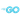# Slices in Go

In Go, a `slice` is a data structure representing data in a sequence. In Go, it is described by:

• A pointer to the first element of the an underlying array
• The length
• The capacity

Here we have two slices: `s` and `s2`.

``````func main() {
var s []int
fmt.Printf("Type of s: %T\n", s)   // Type of s: []int
fmt.Printf("Value of s: %v\n", s)  // Value of s: []

s2 := []int{1, 2, 3, 4, 5, 6}
fmt.Printf("Type of s2: %T\n", s2)  // Type of s2: []int
fmt.Printf("Value of s2: %v\n", s2) // Value of s2: [1 2 3 4 5 6]
}``````

A handy way to visualize slices is using `fmt.Printf` with the `#` option with the `%v` verb. `#` modifies the output of the `%v` format specifiers and formats the output to print the literal syntax used to define the variable in Go.

For slices and arrays, this would be the individual elements of the array/slice along with their types.

``````func main() {
var s []int
fmt.Printf("Value of s: %#v\n", s)  // Value of s: []int(nil)

s2 := []int{1, 2, 3, 4, 5, 6}
fmt.Printf("Value of s2: %#v\n", s2) // Value of s2: []int{1, 2, 3, 4, 5}
}``````

## Slice Mechanics: Creation and Modification

However, we need to understand the implications that slices store a reference to an underlying array.

For example - when a new slice is created by the slice operator the operator returns a new slice, but the new slice points to the same underlying array as the original slice.

``````func main() {
s2 := []int{1, 2, 3, 4, 5, 6}
fmt.Printf("Value of s2: %#v\n", s2) // Value of s2: []int{1, 2, 3, 4, 5}

s3 := s2[1:4]
fmt.Printf("Value of s3: %#v\n", s3) // Value of s3: []int{2, 3, 4}
}``````

We can confirm that the two slices point to the same array by modifying the new slice, `s3`.

``````func main() {
s2 := []int{1, 2, 3, 4, 5, 6}
s3 := s2[1:4]
fmt.Printf("Value of s3: %#v\n", s3) // Value of s3: []int{2, 3, 4}

s3 = append(s3, 100)
fmt.Printf("Value of s3: %#v\n", s3) // Value of s3: []int{2, 3, 4, 100}
}``````

When we print out the original `s2`, we'll see that it has also been modified.

``````	fmt.Printf("Value of s2: %#v\n", s2) // Value of s2: []int{1, 2, 3, 4, 100, 6}
^^^``````

If you remember from this article: Basic Data Structures in Go, we can visualize how the new slice points to the same underlying array as the original slice.

``````s2 := []int{1, 2, 3, 4, 5, 6}
s3 := s2[1:4]

s2
ptr   len   cap
+-----+-----+-----+
|  +  |  6  |  6  | []int
+--|--+-----+-----+
|
v
+-----+-----+-----+-----+-----+-----+
|  1  |  2  |  3  |  4  |  5  |  6  | int
+-----+-----+-----+-----+-----+-----+
▲           ▲
|           |
+------+-----------+
|
s3   |
ptr   len   cap
+-----+-----+-----+
|  +  |  3  |  5  | []int
+-----+-----+-----+``````

Because `s3` points to the same underlying array as `s2`, when we modify `s3`, we are also modifying `s2`.

``````s2 := []int{1, 2, 3, 4, 5, 6}
s3 := s2[1:4]
s3 = append(s3, 100)

s2
ptr   len   cap
+-----+-----+-----+
|  +  |  6  |  6  | []int
+--|--+-----+-----+
|
v
+-----+-----+-----+-----+-----+-----+
|  1  |  2  |  3  |  4  | 100 |  6  | int
+-----+-----+-----+-----+-----+-----+
▲                 ▲
|                 |
+------+-----------------+
|
s3   |
ptr   len   cap
+-----+-----+-----+
|  +  |  4  |  5  | []int
+-----+-----+-----+

// Value of s3: []int{2, 3, 4, 100}
// Value of s2: []int{1, 2, 3, 4, 100, 6}
^^^``````

## Passing Slices as Function Arguments

In Go, although functions arguments are "pass by copy", we need to pay attention when passing slices to functions because it is actually a copy of the "slice header" that is being passed.

This means that the copied slice header is still pointing to the same underlying array, and if we modify the slice within the function, we will --also-- modify the original slice.

For example, here we have a function `median` that takes a slice of `float64` values and returns the median value.

``````func median(values []float64) float64 {
sort.Float64s(values)

i := len(values) / 2
if len(values)%2 == 1 {
return values[i]
}

return (values[i-1] + values[i]) / 2
}``````

However, after passing in the original slice, we see that `vs` is also modified and the original values are now sorted.

``````func main() {
vs := []float64{2, 1, 3, 4}
fmt.Println("Median: ", median(vs))
fmt.Println("Original slice:", vs)
}
// Median: 2.5
// Original slice: [1 2 3 4]``````

This is because the copy we receive in the function is a copy of the slice header, but it still points to the same underlying array.

To fix this, we need to create a new slice and copy the values from the original slice into the new slice.

``````func median(values []float64) float64 {
// Create new slice to not change values
nums := make([]float64, len(values))
copy(nums, values)

sort.Float64s(nums)

i := len(nums) / 2
if len(nums)%2 == 1 {
return nums[i]
}

return (nums[i-1] + nums[i]) / 2
}``````